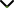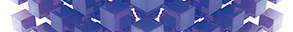# New Trends for Differential Equations with Engineering Applications

Publishing date
01 Feb 2023
Status
Closed
07 Oct 2022

Guest Editors

1Mansoura University, Mansoura, Egypt

2Bangalore University, Bengaluru, India

This issue is now closed for submissions.

# New Trends for Differential Equations with Engineering Applications

This issue is now closed for submissions.

## DescriptionDifferential equations can be considered the most important tool for mathematical modeling and understanding the complicated dynamics of several important real-world problems which arise in engineering, mechanics, physics, chemistry, agriculture, infectious diseases, ecology, neuronal networks, optics, nanophotonics, economics, and finance.

On one side, the search for efficient and reliable computational and analytical solution techniques for conventional differential equations, fractional-order differential equations, and functional differential equations captures the interest of many researchers and mathematicians. On the other side, developing bifurcation methods and theories of dynamical systems for exploring the influences of parameters on qualitative behaviors of differential equations is another focal point of increasing importance.

This Special Issue welcomes original research and review articles of exceptional merit containing novel state-of-the-art computational or analytical techniques for solving/analysis of differential equations in addition to research results having possible applications in science and engineering.

Potential topics include but are not limited to the following:

• Integer and fractional order initial/boundary value problems
• Mathematical models with partial differential equations
• Integer and fractional-order differential equations
• Artificial neural network techniques and applications
• Exact solution techniques for differential equations
• Applications to real-world phenomena
• Fixed point theory and applications to differential equations
• Applied bifurcation methods for dynamical systems
• Chaotic systems and their applicationsJournal metrics
See full report
Acceptance rate43%
Submission to final decision54 days
Acceptance to publication27 days
CiteScore2.100
Journal Citation Indicator-
Impact Factor-Author guidelinesEditorial boardDatabases and indexing

Article of the Year Award: Outstanding research contributions of 2021, as selected by our Chief Editors. Read the winning articles.